aov()函数的语法为aov(formula,data=dataframe),formula可使用的特殊符号如下，其中y为因变量，A、B、C为自变量。

+ 分隔自变量

* 表示所有可能的交互项，如y～A * B *C等价于y～A+B+C+A:B+A:C+B:C+A:B:C
^ 表示交互项达到的某个次数，如y～(A+B+C)^2等价于y～A+B+C+A:B+A:C+B:C
. 表示包含除因变量以外的所有变量。如y～.

## 单因素方差分析（one-way ANOVA）

a <- c(45, 44, 43, 47, 48, 44, 46, 44, 40, 45, 42, 40, 43, 46, 47, 45,
46, 45, 43, 44)
b <- c(45, 48, 47, 43, 46, 47, 48, 46, 43, 49, 46, 43, 47, 46, 47, 46,
45, 46, 44, 45, 46, 44, 43, 42, 45)
c <- c(47, 48, 45, 46, 46, 44, 45, 48, 49, 50, 49, 48, 47, 44, 45, 46,
45, 43, 44, 45, 46, 43, 42)
dfCRp <- data.frame(length = c(a, b, c), site = factor(c(rep("1", 20),
rep("2", 25), rep("3", 23))))
boxplot(length ~ site, data = dfCRp, xlab = "Sites", ylab = "Length")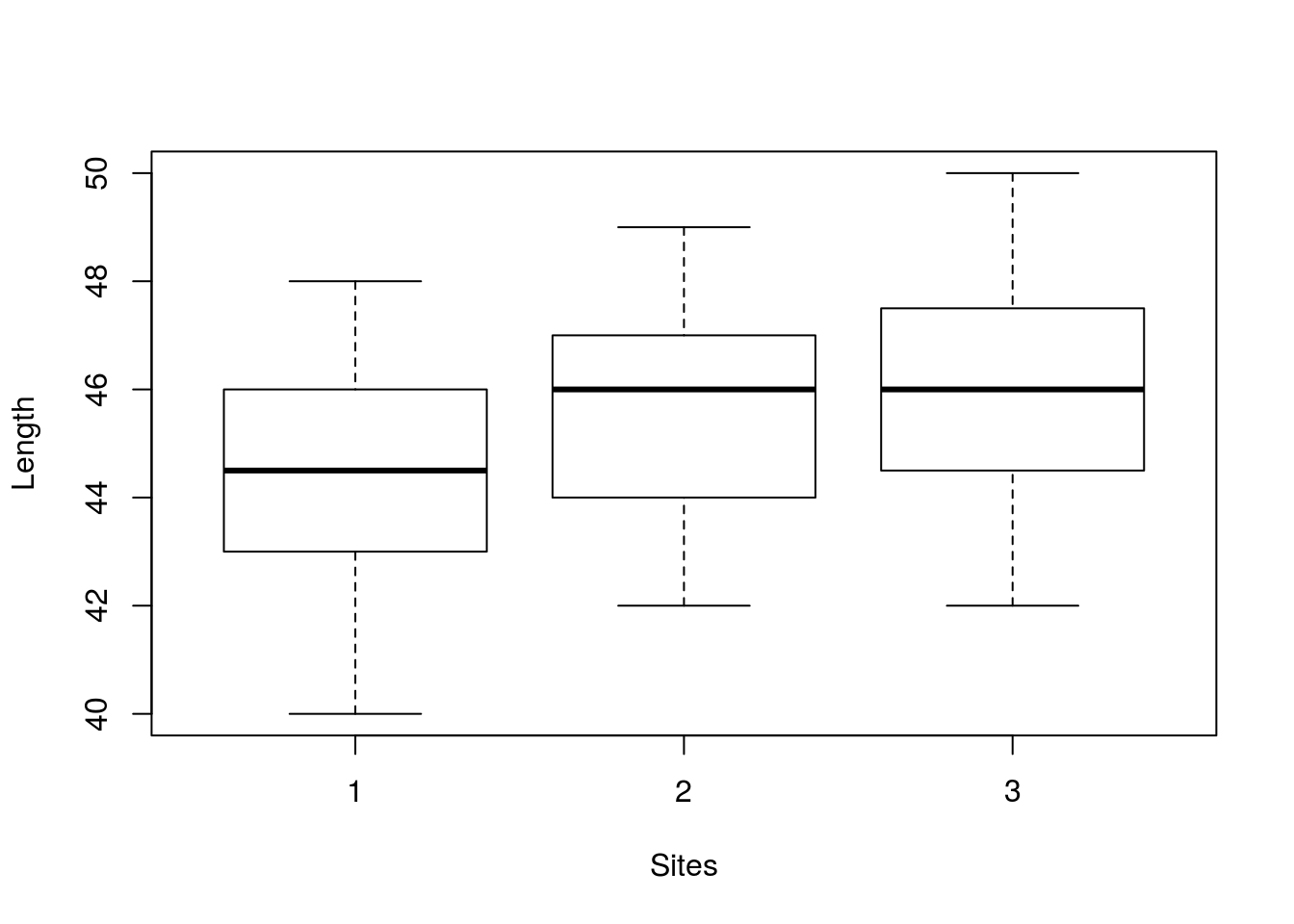plot.design(length ~ site, fun = mean, data = dfCRp, main = "Group means")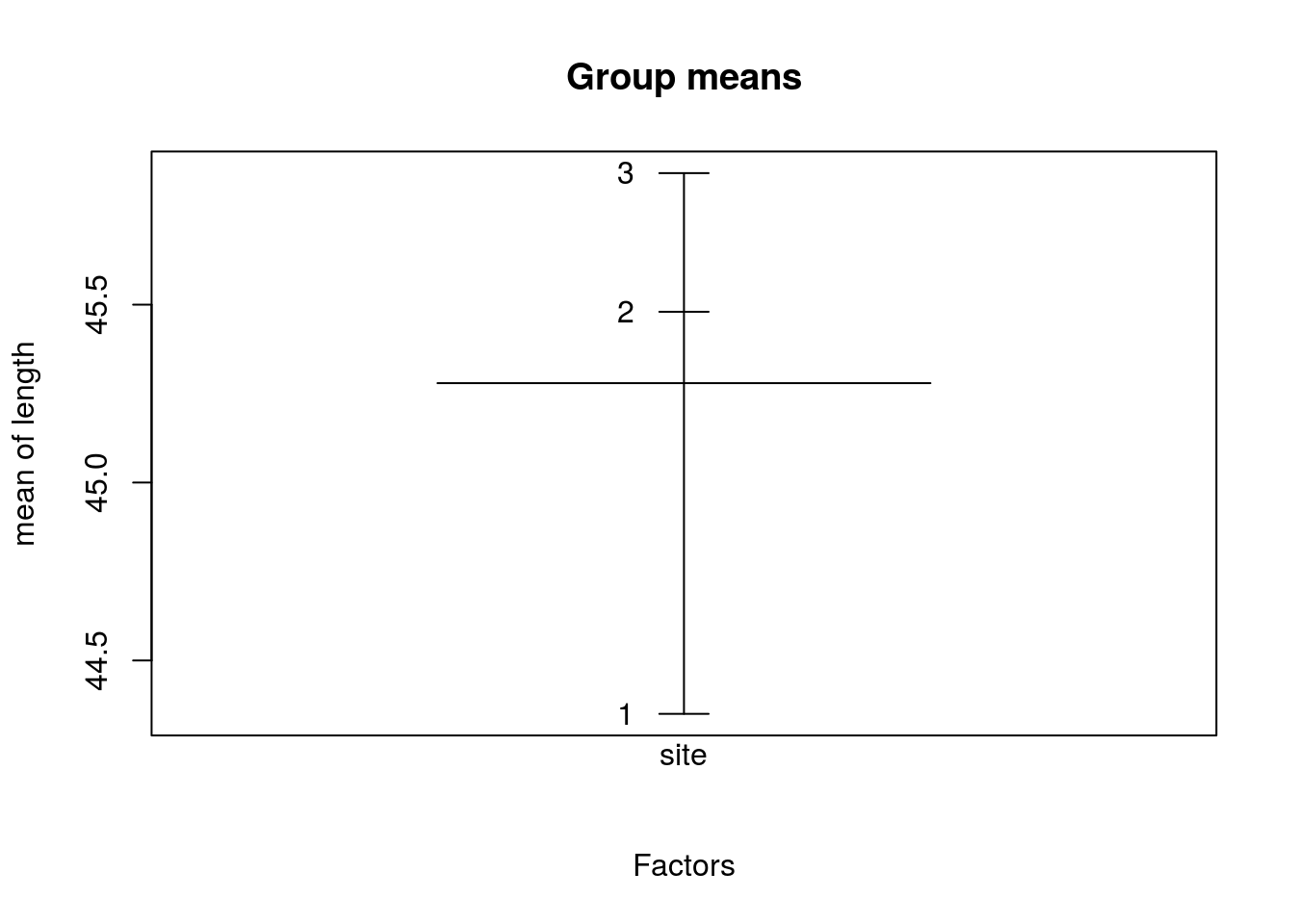箱形图中可观察到不同的因素对于因变量的影响。

### 假设检验

shapiro.test(dfCRp$length) ## ## Shapiro-Wilk normality test ## ## data: dfCRp$length
## W = 0.97397, p-value = 0.1654
bartlett.test(length ~ site,data = dfCRp) #Fligner-Killeen(fligner.test()函数)和Brown-Forsythe检验(HH包中的hov()函数)也可以用来检验方差齐性
##
##  Bartlett test of homogeneity of variances
##
## data:  length by site
## Bartlett's K-squared = 0.76406, df = 2, p-value = 0.6825

### oneway.test()和aov()函数进行方差分析

aovCRp =aov(length ~ site, data = dfCRp)
summary(aovCRp)
##             Df Sum Sq Mean Sq F value Pr(>F)
## site         2  26.29  13.146   3.244 0.0454 *
## Residuals   65 263.40   4.052
## ---
## Signif. codes:  0 '***' 0.001 '**' 0.01 '*' 0.05 '.' 0.1 ' ' 1
#oneway.test(length ~ site, data=dfCRp, var.equal=TRUE)，与aov()结果基本相同。
plotmeans(length ~ site,data =dfCRp ) #绘制有置信区间的组均值图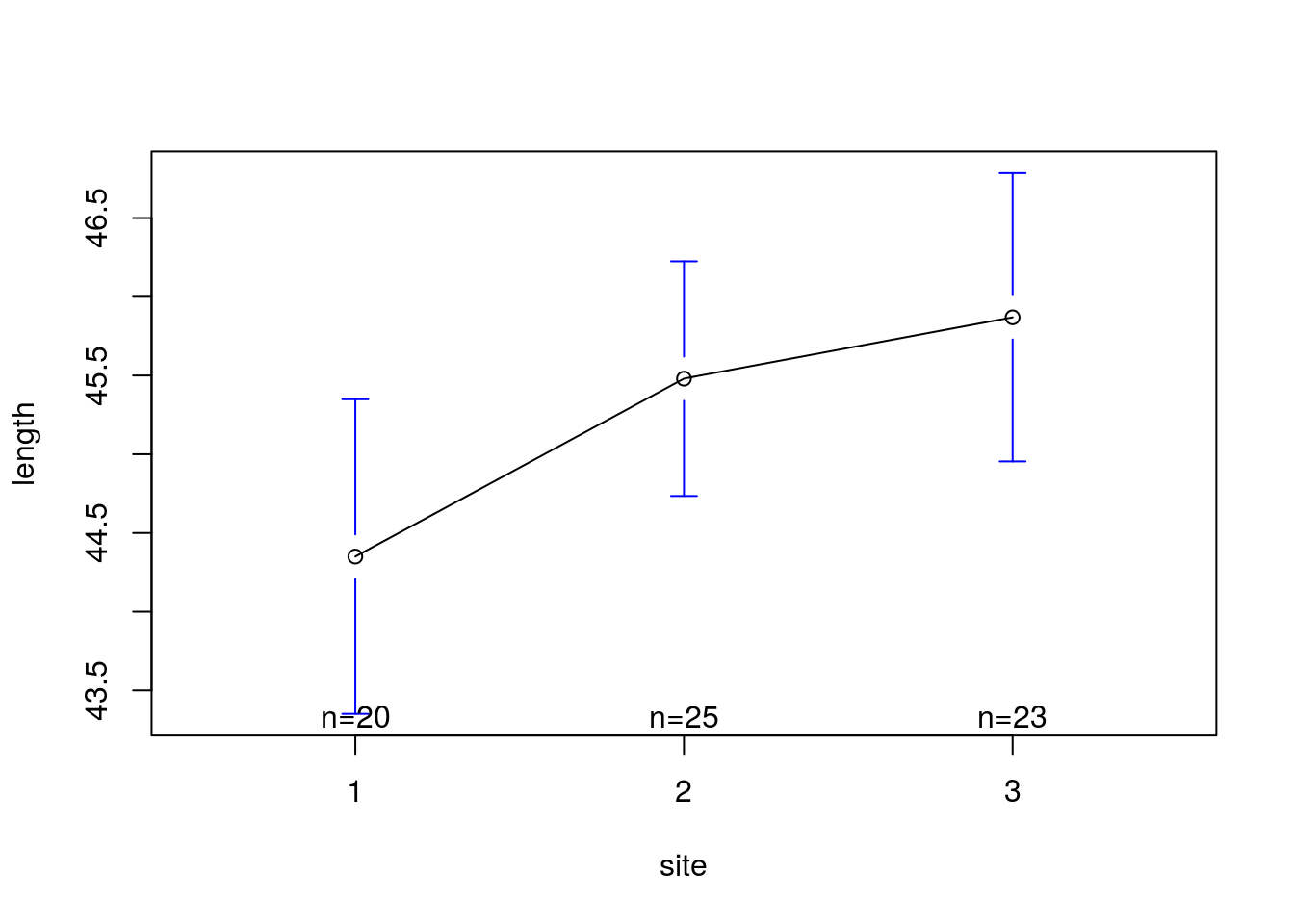par(mfrow=c(2,2))
plot(aovCRp)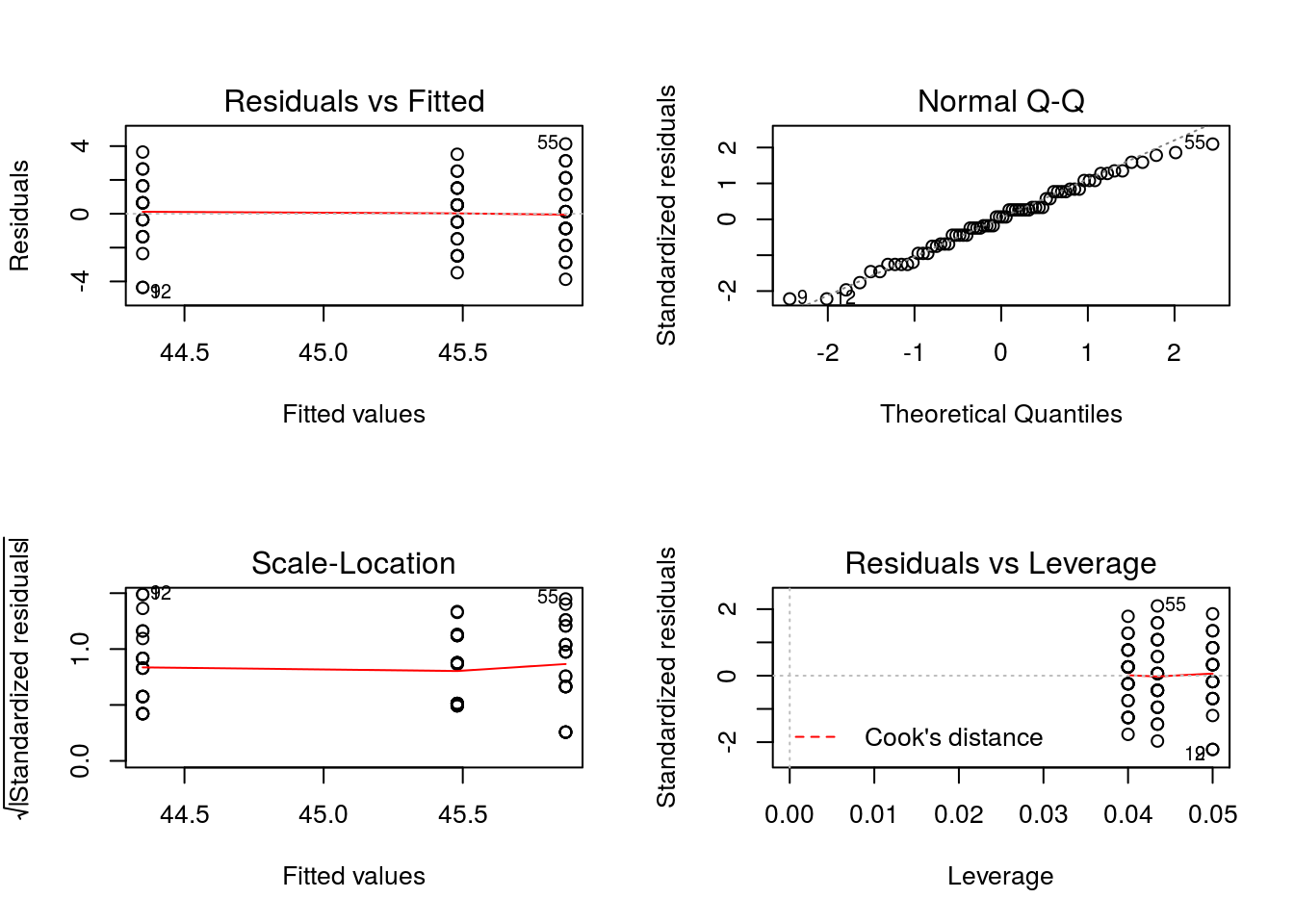par(mfrow=c(1,1))

### 模型比较

(anovaCRp <- anova(lm(length ~ site, data=dfCRp)))
## Analysis of Variance Table
##
## Response: length
##           Df  Sum Sq Mean Sq F value Pr(>F)
## site       2  26.292 13.1462  3.2442 0.0454 *
## Residuals 65 263.399  4.0523
## ---
## Signif. codes:  0 '***' 0.001 '**' 0.01 '*' 0.05 '.' 0.1 ' ' 1
anova(lm(length ~ 1, data=dfCRp), lm(length ~ site, data=dfCRp))
## Analysis of Variance Table
##
## Model 1: length ~ 1
## Model 2: length ~ site
##   Res.Df    RSS Df Sum of Sq      F Pr(>F)
## 1     67 289.69
## 2     65 263.40  2    26.293 3.2442 0.0454 *
## ---
## Signif. codes:  0 '***' 0.001 '**' 0.01 '*' 0.05 '.' 0.1 ' ' 1
anovaCRp["Residuals", "Sum Sq"]
##  263.3987

### 效果大小(Effect size)

dfSSb <- anovaCRp["site",        "Df"]
SSb   <- anovaCRp["site",        "Sum Sq"]
MSb   <- anovaCRp["site",        "Mean Sq"]
SSw   <- anovaCRp["Residuals", "Sum Sq"]
MSw   <- anovaCRp["Residuals", "Mean Sq"]

(etaSq <- SSb / (SSb + SSw))#   DescTools包中EtaSq(aovCRp, type=1)函数可以计算
##  0.09076038
(omegaSq <- dfSSb * (MSb-MSw) / (SSb + SSw + MSw)) 
##  0.06191765
(f <- sqrt(etaSq / (1-etaSq)))
##  0.3159432

${\eta }^{2},{\omega }^{2},{f}^{2}$$\eta^{2},\omega^{2},f^{2}$值如上，如${\eta }^{2}$$\eta^{2}$实验处理之后各组间平方和在总体平方和中所占的比重，${\eta }^{2}$$\eta^{2}$越大反映实验效果大。一般${\eta }^{2}$$\eta^{2}$大于0.14，就认为效果有大的效果。

### 多重比较

cntrMat <- rbind("a-c"          =c(1,0,-1),
"1/3*(a+b)-c"=c(1/3,1/3,-1),
"b-c"          =c(0,1,-1))
summary(glht(aovCRp, linfct=mcp(site=cntrMat), alternative="less"),
test=adjusted("none"))
##
##   Simultaneous Tests for General Linear Hypotheses
##
## Multiple Comparisons of Means: User-defined Contrasts
##
##
## Fit: aov(formula = length ~ site, data = dfCRp)
##
## Linear Hypotheses:
##                  Estimate Std. Error t value  Pr(<t)
## a-c >= 0          -1.5196     0.6155  -2.469 0.00809 **
## 1/3*(a+b)-c >= 0  -1.1429     0.5331  -2.144 0.01790 *
## b-c >= 0          -0.3896     0.5816  -0.670 0.25268
## ---
## Signif. codes:  0 '***' 0.001 '**' 0.01 '*' 0.05 '.' 0.1 ' ' 1
## (Adjusted p values reported -- none method)
#pairwise.t.test(dfCRp$length, dfCRp$site, p.adjust.method="bonferroni") #结果与glht()函数类似。

#### 非计划的多重比较(Planned comparisons - post-hoc)

#ScheffeTest检验
ScheffeTest(aovCRp, which="site", contrasts=t(cntrMat))  #DescTools包
##
##   Posthoc multiple comparisons of means : Scheffe Test
##     95% family-wise confidence level
##
## $site ## diff lwr.ci upr.ci pval ## 1-3 -1.5195652 -3.061467 0.02233664 0.0543 . ## 1,2-3 -15.9262319 -17.092478 -14.75998618 <2e-16 *** ## 2-3 -0.3895652 -1.846661 1.06753062 0.7997 ## ## --- ## Signif. codes: 0 '***' 0.001 '**' 0.01 '*' 0.05 '.' 0.1 ' ' 1 #Tukey HSD检验 (tHSD <- TukeyHSD(aovCRp)) ## Tukey multiple comparisons of means ## 95% family-wise confidence level ## ## Fit: aov(formula = length ~ site, data = dfCRp) ## ##$site
##          diff         lwr      upr     p adj
## 2-1 1.1300000 -0.31850529 2.578505 0.1552673
## 3-1 1.5195652  0.04333482 2.995796 0.0422495
## 3-2 0.3895652 -1.00547115 1.784602 0.7817904
plot(tHSD)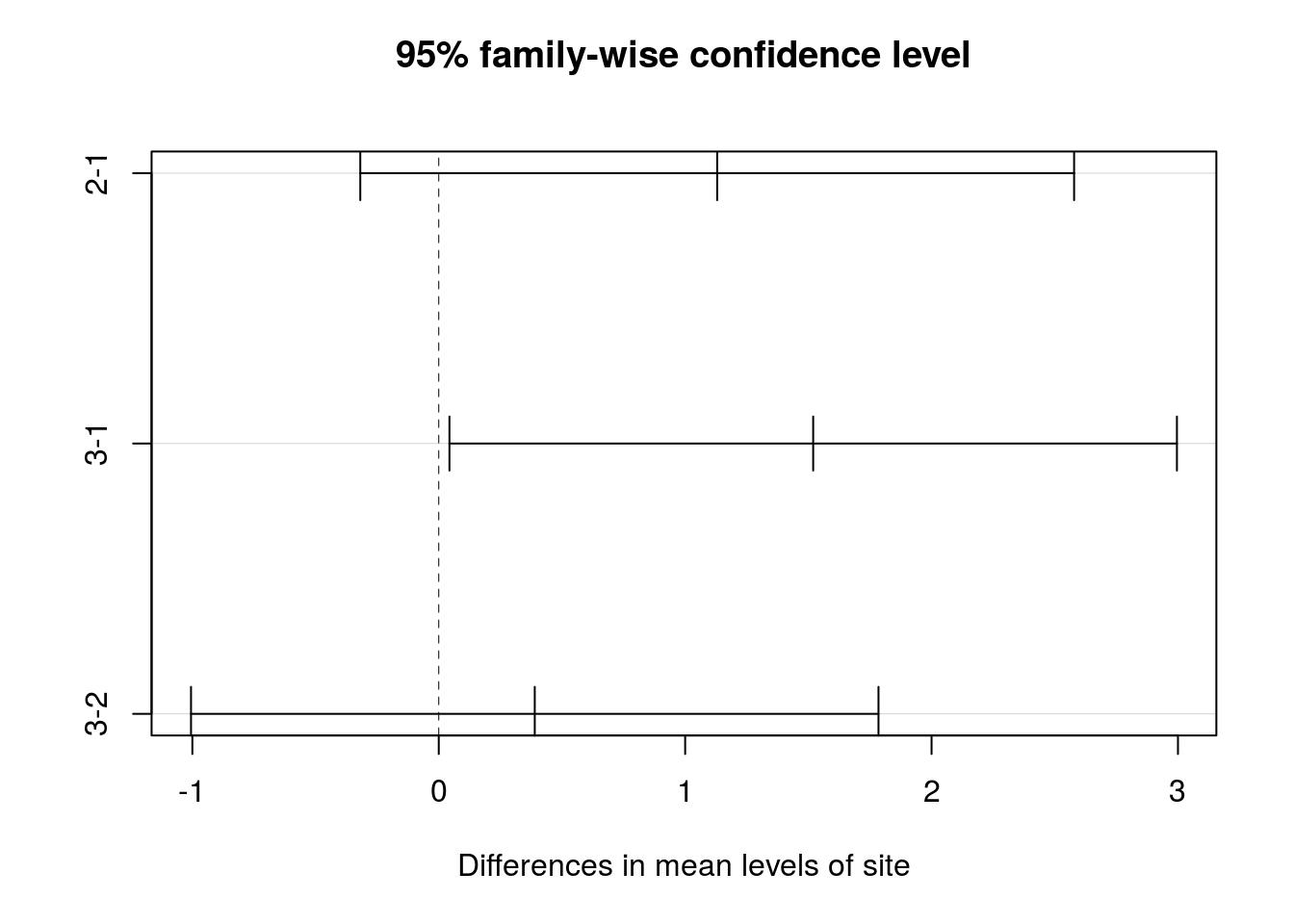置信区间包含0说明差异不显著。

multcomp包中glht()函数提供了多重均值更全面的方法，适用于线性模型和广义线性模型。下面的代码重现Tukey HSD检验。

tukey <- glht(aovCRp, linfct=mcp(site="Tukey"))
summary(tukey)
##
##   Simultaneous Tests for General Linear Hypotheses
##
## Multiple Comparisons of Means: Tukey Contrasts
##
##
## Fit: aov(formula = length ~ site, data = dfCRp)
##
## Linear Hypotheses:
##            Estimate Std. Error t value Pr(>|t|)
## 2 - 1 == 0   1.1300     0.6039   1.871   0.1552
## 3 - 1 == 0   1.5196     0.6155   2.469   0.0422 *
## 3 - 2 == 0   0.3896     0.5816   0.670   0.7817
## ---
## Signif. codes:  0 '***' 0.001 '**' 0.01 '*' 0.05 '.' 0.1 ' ' 1
## (Adjusted p values reported -- single-step method)
plot(cld(tukey,level = .05),col="lightgrey") #cld()函数中level选项设置了使用显著水平0.05，即95%的置信区间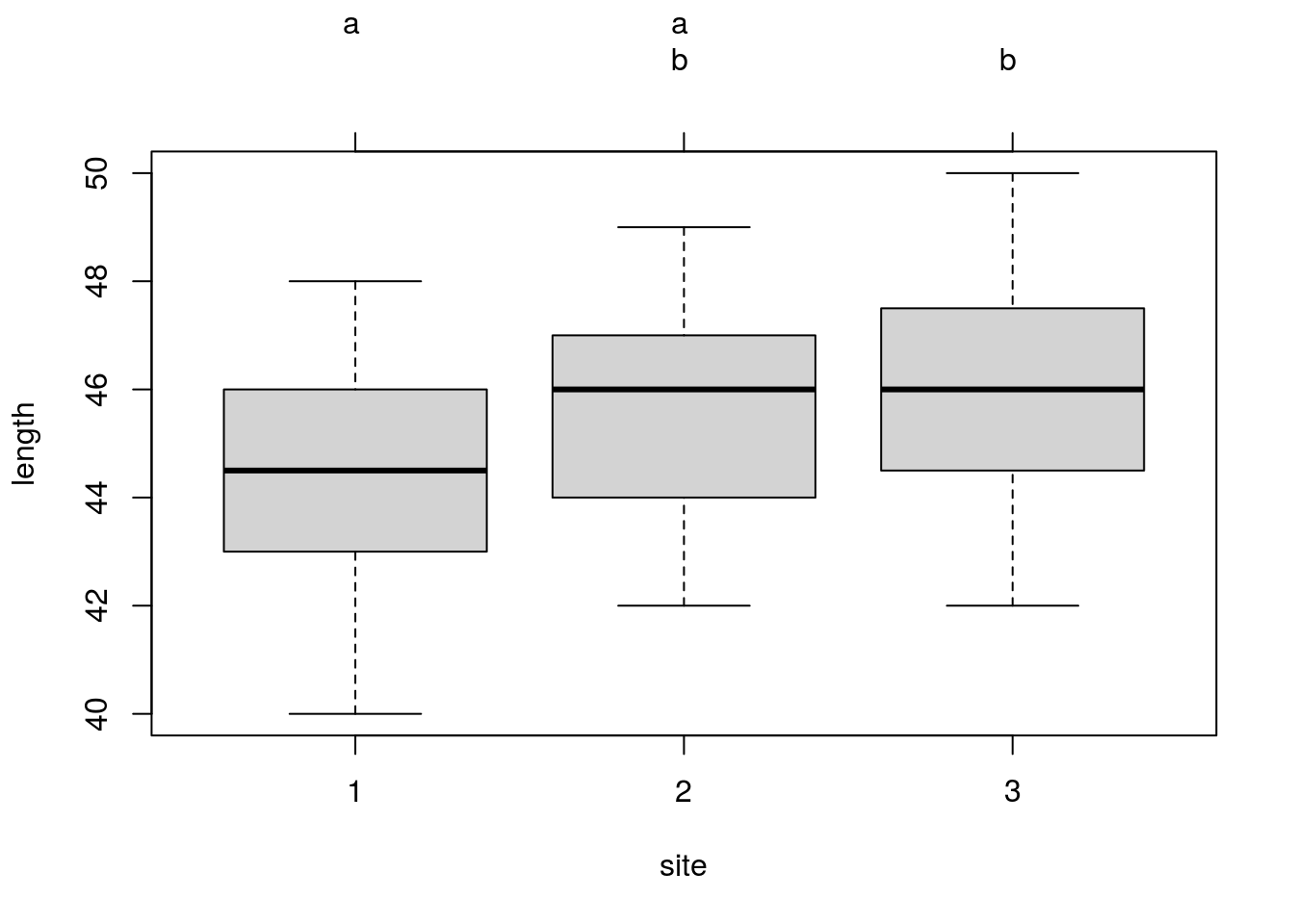有相同字母的组（箱线图表示）说明均值差异不显著。

### 离群点检测

outlierTest(aovCRp)
##
## No Studentized residuals with Bonferonni p < 0.05
## Largest |rstudent|:
##    rstudent unadjusted p-value Bonferonni p
## 9 -2.288156           0.025441           NA

### 残差的相关检验

Estud <- rstudent(aovCRp)
shapiro.test(Estud)
##
##  Shapiro-Wilk normality test
##
## data:  Estud
## W = 0.9876, p-value = 0.7381
qqnorm(Estud, pch=20, cex=2)
qqline(Estud, col="gray60", lwd=2)